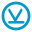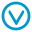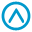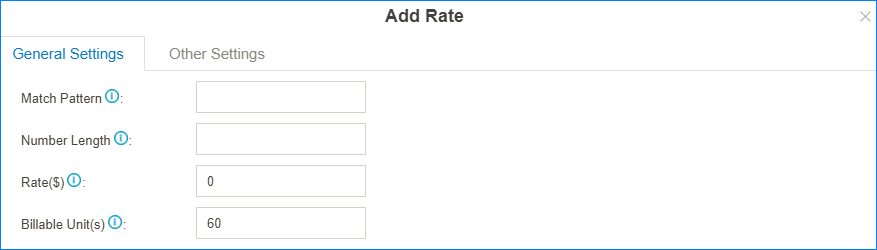# Billing Rate

Set up billing rate according to extensions/accounts, time periods, call duration, call types (whether it’s local or international, from landline or mobile phone).

When calls are made to external numbers, they are checked against the “Match Pattern”, “Number Length”, and “Time”.

System will match the called number with billing rates from top to bottom. You can adjust the rate sequence by clicking these buttons.

The matching priority is as bellow:

1. Time
2. Number Length
3. Match Pattern## Call Costs

If a match is found then the cost is calculated as follows:

• Total Cost = Initial Cost + Billable Unit Number * Rate (less than one billing unit will be regarded as one billing unit).
• If the talking time is less than the “Initial Time”, the Total Cost = Initial Cost.

Below is an example billing rate setting, please check call cost details for different calls.

• Initial Time: 120 seconds
• Initial Cost: 0.2\$
• Rate: 0.3\$
• Billable Unit: 60 seconds
Table 1. Billing Rate setting example
Talk Time (s) Total Cost (\$) Call Cost Details
68 0.2

Talk Time(68) < Initial Time(120)

Total Cost = Initial Cost

125 0.5

Talk Time: 125=120+5

Total Cost: 0.2+0.3*1=0.5

180 0.5

Talk Time: 180=120+60*1

Total Cost: 0.2+0.3*1=0.5

190 0.8

Talk Time: 190=120+60*1+10

Total Cost: 0.2+0.3*2=0.8

380 1.7

Talk Time: 380=120+4*60+20

Total Cost: 0.2+0.3*5=1.7

## Call Rate Settings

Go to Billing > Rate to add or edit billing rate.

General Settings of Rate• Match Pattern: The prefix of the called number. This setting must match the dial pattern of the outbound routes in your PBX. Leave it blank, the rate will apply to all numbers.
Restriction: Wildcard character `.` and `!` are not allowed.
• Number Length: Used to restrict the length of called number. If the length of dialed number is shorter or equal to the “Number Length”, the rate will apply to it. Leave it blank, the rate will apply to all numbers.
• Rate: After the initial time, each billable unit will be charged with this rate.
• Billable Unit: Set the billable unit after initial time. If the rate is \$0.2 and billable unit is 60 seconds, the call you make will cost \$0.2 per 60 seconds (less than one billing unit will be regarded as one billing unit).

Other Settings of Billing Rate• Initial Time & Initial Cost: If the Initial Cost is `\$0.2` and the Initial Time is `60` seconds, it means the first 60 seconds of this call will cost \$0.2.
• Time: Set when the rate will be applied to.
• Days of Week: Set which days will the rate applies to.
• Member Extensions: Choose which extensions will the rate applies to.
• Member Accounts: Choose which accounts will the rate applies to.

## Import/Export Call Rates

You can import and export rate rules; this helps you manage billing rates easily.

Go to Billing > Rate to import or export the call rates.

• Click Import to import a guest list
Tip: You can export a guest file from PBX and use it as a sample to start with.
• Click Export to export the guests into a `csv` file